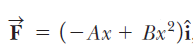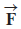# Problem: A single conservative force acting on a particle within a system varies as , where A and B are constants,  is in newtons, and x is in meters. (a) Calculate the potential energy function U(x) associated with this force for the system, taking  U = 0 at x = 0. Find (b) the change in potential energy and (c) the change in kinetic energy of the system as the particle moves from x = 2.00 m to x = 3.00 m.

###### FREE Expert Solution

This problem involves the relationship between potential energy and force.

Force:

79% (470 ratings)###### Problem Details

A single conservative force acting on a particle within a system varies as, where A and B are constants,is in newtons, and x is in meters. (a) Calculate the potential energy function U(x) associated with this force for the system, taking  U = 0 at x = 0. Find (b) the change in potential energy and (c) the change in kinetic energy of the system as the particle moves from x = 2.00 m to x = 3.00 m.